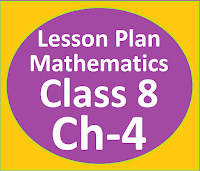## Posts

Showing posts from July, 2023

### Resource Centre Mathematics

Resource Centre Mathematics Mathematics worksheet, mathematics basic points and formulas, mathematics lesson plan, mathematics multiple choice questions Workplace Dashboard CBSE Syllabus For Session 2023-24 For  :   Classes IX & X    |   Classes XI & XII Watch Videos on Maths Solutions CLASS IX MATHEMATICS FORMULAS &  BASIC CONCEPTS

### Lesson Plan Math Class 8 | Direct and Inverse Variation CH-4E- LESSON PLAN   SUBJECT MATHEMATICS    CLASS- 8 Lesson Plan for CBSE mathematics class 8 Direct & Inverse Variation, Step by step teaching strategy for mathematics teachers. Perfect lesson plan which makes the teaching learning process perfect